# Fundamentals projective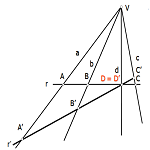An axiomatic logical system of the definition of a small number of basic elements that are related by a set of rules. Applying these rules to infer properties or theorems which in turn are useful for generating new properties.

It thus generates a rich set of relationships from basic definitions (elements) and a limited number of rules (axioms).

Inference rules are projective operations that relate to items.

The basic elements of classical geometry are the points, straight and flat. You can define new geometries with different elements and other basic operations and axioms.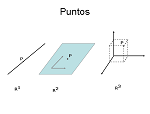These elements are the numbers of the geometry, and can be used, along with graphical transformations as operators, to idealize and describe models of reality.

The items belong to one-dimensional space, two-dimensional, three-dimensional …

The geometric figures are formed from the basic elements, so interested in studying possible relations. In particular the invariant based approach leads to a mindset that groups the concepts according to their independence in the application to solving problems.

The simple reason and the cross ratio, as will be seen later, enable new models of solution in problems that have been dealt normally from a Pythagorean perspective, in which the absolute measurements of the distances, rather its relations with other, constitute the argument element.

Operating with these items, groups remaining to be considered early. For example, if the base element is the point, the line and the plane must be reduced to sets of points; the line should be understood as an infinite set of points that can be determined with a simple parameter or coordinate with respect to a point source,; plane as a set of infinite points determined by two parameters of a coordinate system.

This way of linking the elements allows us to generalize the theorems obtained for a given element, the other elements.

The ley duality recoge this idea by providing a mechanism for this purpose semantic. A simple change of words in a theorem on straight, you can become one for flat or logical abstraction points by simplifying the geometric structure.

## Elements and Geometric Shapes

Los geometric elements can structure containing groups and designated geometric shapes.

For example, elements (points) three-dimensional space R3 distance that a constant length of another die determined the geometric shape called sphere. The equidistant from a line determine a cylinder or cylindrical surface of revolution, and which are equidistant from a plane formed two planes parallel to the above new, etc..

You can use a semantics that generalizes the concepts regardless of the nature of the constituent basic elements. Note the "dual form" in which the following statements are presented.

 A line can be understood as a basic geometric element or as a infinite set of points.In this second case we will say that The base line is geometric shape called "straight Series". A point can be treated as a basic geometric element or as the infinite straight intersection.In this second case we will say that point is the vertex (base) geometric form called "Make straight".

The "straight Series", "Make straight" and "Make planes" are the most basic geometric shapes. Its elements are points, lines and planes respectively.

 Rectilinear Series The infinite points form a straight.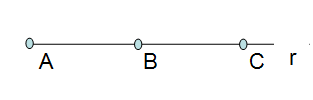Make straight What are the lines of equal infinite vertex.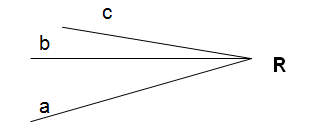Make plans The form infinite planes share a line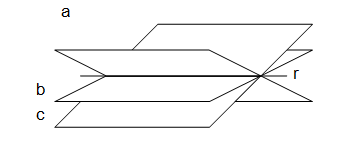Table 1 Forms notch